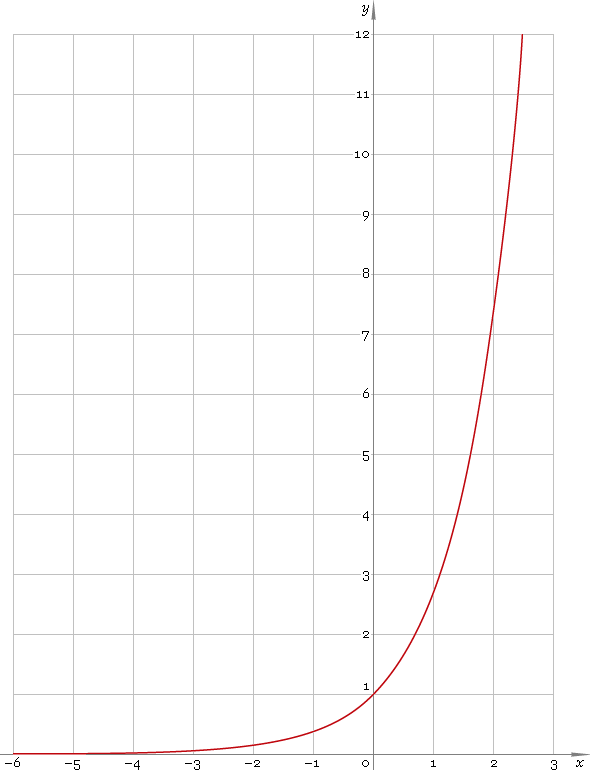The Art of Mathematics

# exp — exponential function

## 1. Definition

Exponential function is the function of kind

ex

where constant e is selected so that slope of the function at point x = 1 is 45°.

## 2. Graph

Exponential function is defined everywhere on real axis. Its graph is depicted below — fig. 1.Fig. 1. Graph of the exponential function y = ex.

Function codomain is positive half of the real axis: (0, +∞).

## 3. Identities

By definition:

elnxx

Negative argument:

ex = 1 /ex

Sum and difference of arguments:

ex + y = ex ey
exy = ex/ey

Product of arguments:

exy = (ex)y

Base change:

ax = ex lna

## 4. Derivative and indefinite integral

Exponent derivative:

ex′ = ex

Indefinite integral of the exponent:

∫ ex dx = ex + C

where C is an arbitrary constant.

## 5. How to use

To calculate exponent of the number:

``exp(−1);``

To get exponent of the complex number:

``exp(−1+i);``

To get exponent of the current result:

``exp(rslt);``

To get exponent of the number z in calculator memory:

``exp(mem[z]);``

## 6. Support

Exponent of the real argument is supported in free version of the Librow calculator.

Exponent of the complex argument is supported in professional version of the Librow calculator.